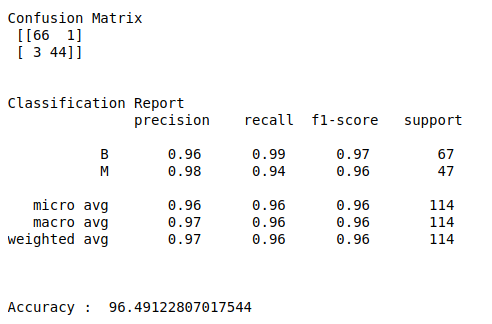• #5, First Floor, 4th Street Dr. Subbarayan Nagar Kodambakkam, Chennai-600 024 Landmark : Samiyar Madam
• pro@slogix.in
• +91- 81240 01111

#### Social List

##### Detecting breast cancer using KNN algorithm in python?
###### Description

To train a machine learning model for detect breast cancer using KNN in python.

#### Input

Breast cancer data set. (Kaggle)

#### Output

Confusion matrix, classification report and accuracy_score.

###### Process

Import library.

Declare independent and dependent variables.

Do pre process for text data.

Split the data into train and test.

Build the model.

Fit the train data into the model.

Evaluate the model using test data.

###### Sapmle Code

#import libraries
import pandas as pd
import numpy as np
import matplotlib.pyplot as plt
import seaborn as sns
from sklearn import preprocessing
from sklearn.preprocessing import Normalizer
from sklearn.model_selection import train_test_split
from sklearn.model_selection import GridSearchCV
from sklearn.neighbors import KNeighborsClassifier
from sklearn.metrics import classification_report, confusion_matrix, accuracy_score

#check missing values
print(“Checking missing values\n”)
print(data.isnull().sum())

#make it as a data frame
df = pd.DataFrame(data)
print(“\n”)

#print data shape
print(“Shape of data\n”,df.shape)

#counts in each class
print(“\n”)
print(“Counts in each class\n”)
count = df[‘diagnosis’].value_counts()
print(count)

#Count plot for target
plt.rcParams[“figure.figsize”] = [9,6]
sns.countplot(x=’diagnosis’,hue=”diagnosis”, data=df)
plt.show()

#Define X and y variable
X = df.iloc[:,2:32]
y = df.iloc[:,1]

#Split train and test data
X_train, X_test, y_train, y_test = train_test_split(X, y, test_size=0.2, random_state=0)

#training set and testing set
print(“\n”)
print(“Training data set\n”,X_train,”\n”,y_train)
print(“\n”)
print(“Testing data set\n”,X_test)

#Build the KNN model
knn = KNeighborsClassifier()
#create a dictionary of all values we want to test for n_neighbors
params_knn = {‘n_neighbors’: np.arange(1, 25)}
#use gridsearch to test all values for n_neighbors
knn_gs = GridSearchCV(knn, params_knn, cv=5)
#fit model to training data
knn_gs.fit(X_train, y_train)
knn_best = knn_gs.best_estimator_
y_pred = knn_best.predict(X_test)

#classification report & confusion matrix
print(“Confusion Matrix\n”,confusion_matrix(y_test,y_pred))
print(“\n”)
print(“Classification Report\n”,classification_report(y_test,y_pred))
print(“\n”)
print(“Accuracy : “,accuracy_score(y_test,y_pred)*100)

###### Screenshots